#Chapter 16Preprocessors## 16.2 Eqn

Eqn is a preprocessor designed to facilitate the typesetting of mathematical equations. Use neqn with nroff. Eqn has the following command-line syntax:

`/usr/ucb/eqn` [`options`] [`files`]

Options
-d`xy`

Use `x` and `y` as start and stop delimiters; same as specifying the eqn directive `delim``xy`.

-f`n`

Change to font `n`; same as the `gfont` directive.

-p`n`

Reduce size of superscripts and subscripts by `n` points. If `-p` is not specified, the default reduction is 3 points.

-s`n`

Reduce the point size by `n` points; same as the `gsize` directive.

-T`dev`

Format output to device `dev`. The default value comes from the TYPESETTER environment variable. Not available with neqn.

### 16.2.1 Eqn Macros

.EQ

Start typesetting mathematics.

.EN

End typesetting mathematics.

Use the `checkeq` command to check for unmatched macro pairs.

### 16.2.2 Mathematical Characters

The character sequences below are recognized and translated as shown:

CharacterTranslationCharacterTranslation
>=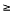approx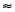<=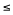nothing
==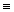cdot.
!=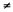times×
+-±del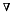->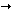grad<-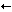......
<<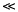,...,,...,
>>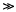sum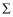inf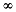int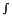partial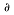prod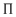half½union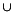prime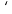inter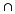### 16.2.3 Mathematical Text

Digits, parentheses, brackets, punctuation marks, and the following mathematical words are printed out in Roman font:

```sin     cos     tan     arc
sinh    cosh    tanh
and     if      for     det
max     min     lim
log     ln      exp
Re      Im```

### 16.2.4 Greek Characters

Greek letters can be printed in uppercase or lowercase. To obtain Greek letters, simply spell them out. Some uppercase Greek letters are not supported because they can be specified by an Arabic equivalent (e.g, A for alpha, B for beta).

CharacterTranslationCharacterTranslation
alpha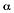tau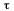beta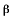upsilon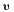gamma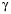phi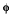delta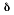chi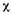epsilon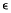psi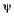zeta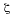omega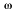eta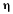GAMMA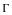theta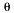DELTA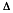iota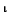THETA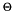kappa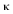LAMBDA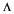lambda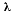XI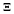mu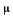PI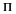nu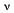SIGMA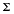xi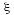UPSILON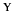omicron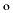PHI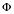pi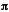PSI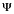rho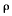OMEGA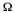sigma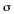### 16.2.5 Diacritical Marks

Several keywords are available to mark the tops of characters. Eqn centers a mark at the correct height. `bar` and `under` will span the necessary length.

CharacterTranslation
x dot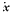x dotdot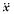x hat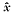x tilde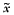x vec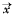x dyad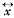x bar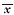x under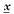### 16.2.6 Keywords Recognized by Eqn

In addition to character names and diacritical marks, eqn recognizes the following keywords:

above

Separate the pieces of a pile or matrix column.

back `n`

Move backwards horizontally `n` 1/100's of an m.

bold

Change to bold font.

ccol

Center align a column of a matrix.

cpile

Make a centered pile (same as pile).

define

Create a name for a frequently used string.

delim `xy`

Define two characters to mark the left and right ends of an eqn equation to be printed in line. Use `delim off` to turn of delimiters.

down `n`

Move down `n` 1/100's of an m.

fat

Widen the current font by overstriking it.

font `x`

Change to font `x`, where `x` is the one-character name or the number of a font.

from

Used in summations, integrals, and similar constructions to signify the lower limit.

fwd `n`

Move forward horizontally `n` 1/100's of an em.

gfont `x`

Set a global font `x` for all equations.

gsize `n`

Set a global size for all equations.

italic

Change to italic font.

lcol

Left justify a column of a matrix.

left

Create big brackets, big braces, big bars, etc.

lineup

Line up marks in equations on different lines.

lpile

Left justify the elements of a pile.

mark

Remember the horizontal position in an equation. Used with lineup.

matrix

Create a matrix.

ndefine

Create a definition which takes effect only when neqn is running.

over

Make a fraction.

pile

Make a vertical pile with elements centered above one another.

rcol

Right adjust a column of a matrix.

right

Create big brackets, big braces, big bars, etc.

roman

Change to roman font.

rpile

Right justify the elements of a pile.

size `n`

Change the size of the font to `n`.

sqrt

Draw a square root sign.

sub

Start a subscript.

sup

Start a superscript.

tdefine

Make a definition which will apply only for eqn.

to

Used in summations, integrals, and similar constructions to signify the upper limit.

up `n`

Move up `n` 1/100's of an em.

~

Force extra space into the output.

^

Force a space one half the size of the space forced by ~ .

{ }

Force eqn to treat an element as a unit.

'...'

A string within quotes is not subject to alterations by eqn.

### 16.2.7 Precedence

If you don't use braces, eqn will do operations in the order shown in this list, reading from left to right.

 dyad vec under bar tilde hat dot dotdot fwd back down up fat roman italic bold size sub sup sqrt over from to

These operations group to the left:

`over   sqrt   left   right`

All others group to the right.

### 16.2.8 Eqn Examples

Input:

```.EQ
delim %%
.EN
%sum from i=0 to inf c sup i~=~lim from {m -> inf}
sum from i=0 to m c sup i%
.EQ
delim off
.EN```

Result: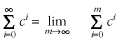Input:

```.EQ
delim %%
.EN
%x ~=~ left [ { -b ~+-~ sqrt {b sup 2 - ~4ac} }
over 2a right ]%
.EQ
delim off
.EN```

Result: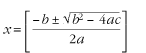16.1 Tbl16.3 Pic